# opencv--图像的基本操作【2】

## 1、图像的表示

1.1、数字图像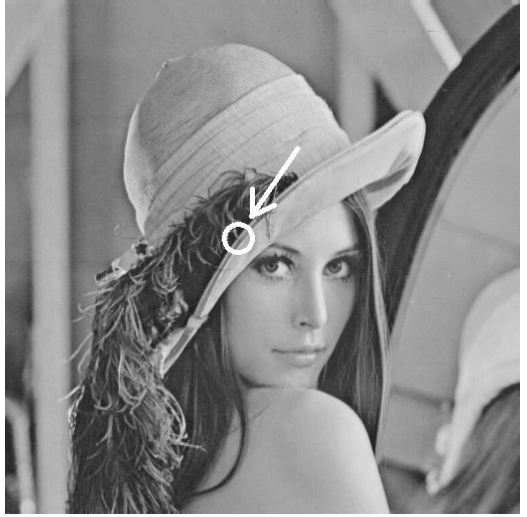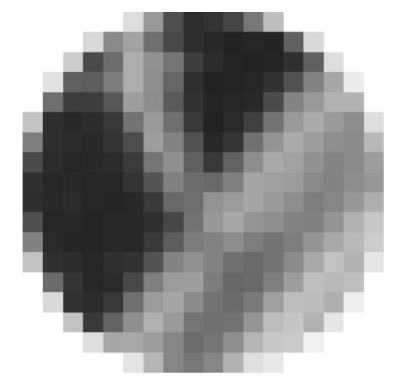1.2、一般来说，灰度图用 2 维矩阵表示，彩色（多通道）图像用 3 维矩阵（M× N × 3）表示。

1.3、图像数据在计算机内存中的存储顺序为以图像最左上点（也可能是最左下点）开始，存储如表所示。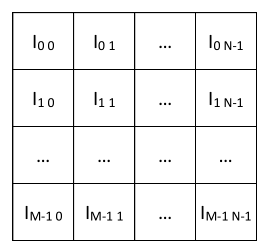I ij 表示第 i 行 j 列的像素值。如果是多通道图像，比如 RGB 图像，则每个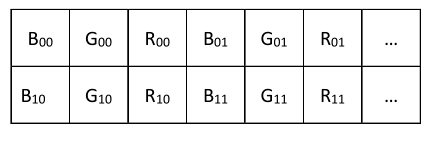# 2、Mat类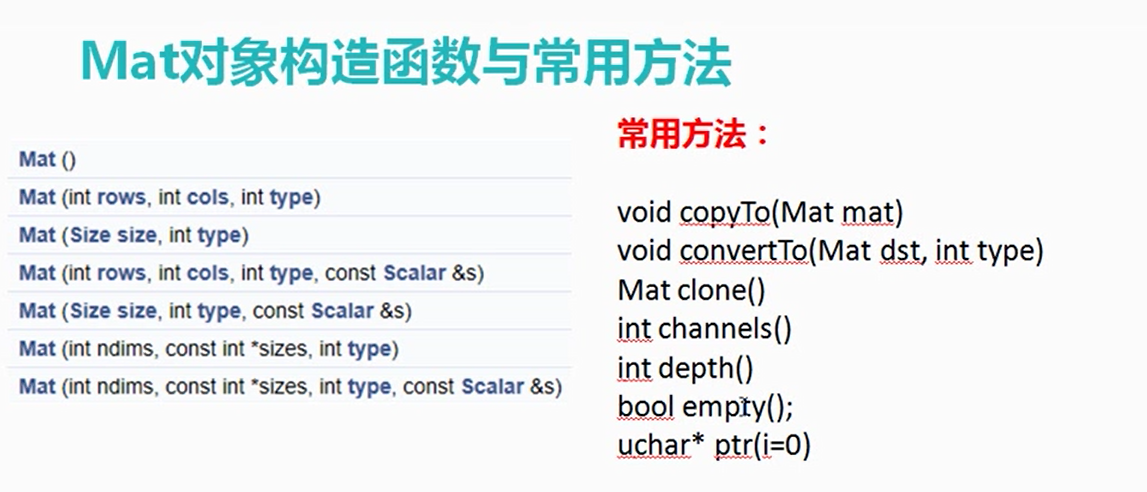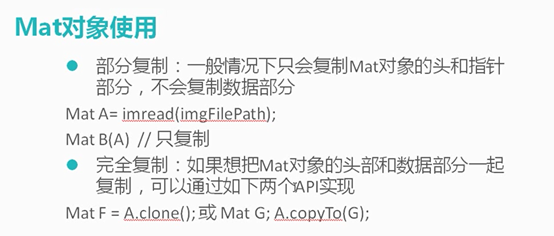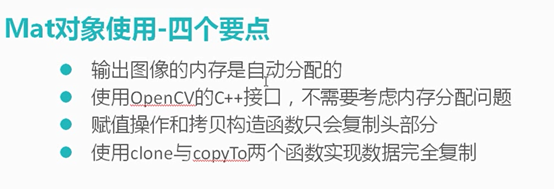## 2.1、Mat 类的定义如下所示，关键的属性如下方代码所示：

class CV_EXPORTS Mat
{
public:
//一系列函数
...
/* flag 参数中包含许多关于矩阵的信息，如：
-Mat 的标识
-数据是否连续
-深度
-通道数目
*/
int flags;
//矩阵的维数，取值应该大于或等于 2
int dims;
//矩阵的行数和列数，如果矩阵超过 2 维，这两个变量的值都为-1
int rows, cols;
//指向数据的指针
uchar* data;
//指向引用计数的指针
//如果数据是由用户分配的，则为 NULL
int* refcount;
//其他成员变量和成员函数
...
};


## 2.2、创建Mat对象

Mat 是一个非常优秀的图像类，它同时也是一个通用的矩阵类，可以用来创建和操作多维矩阵。有多种方法创建一个 Mat 对象。

### 构造函数方法

Mat 类提供了一系列构造函数，可以方便的根据需要创建 Mat 对象。

Mat M(3,2, CV_8UC3, Scalar(0,0,255));
cout << "M = " << endl << " " << M << endl;


• 常用的构造函数有：

Mat::Mat()

Mat::Mat(int rows, int cols, int type)

Mat::Mat(Size size, int type)

Mat::Mat(int rows, int cols, int type, const Scalar& s)

Mat::Mat(Size size, int type, const Scalar& s)

Mat::Mat(const Mat& m)

Mat::Mat(int rows, int cols, int type, void* data, size_t step=AUTO_STEP)

Mat::Mat(Size size, int type, void* data, size_t step=AUTO_STEP)

Mat::Mat(const Mat& m, const Range& rowRange, const Range& colRange)

Mat::Mat(const Mat& m, const Rect& roi)

1. 这些构造函数中，很多都涉及到类型type。type可以是CV_8UC1，CV_16SC1，…，CV_64FC4 等。
2. 里面的 8U 表示 8 位无符号整数，16S 表示 16 位有符号整数，64F表示 64 位浮点数（即 double 类型）；
3. C 后面的数表示通道数，例如 C1 表示一个通道的图像，C4 表示 4 个通道的图像，以此类推。

Mat M(3,2, CV_8UC(5));//创建行数为 3，列数为 2，通道数为 5 的图像

### create()函数创建对象

Mat M(2,2, CV_8UC3);//构造函数创建图像
M.create(3,2, CV_8UC2);//释放内存重新创建图像


### Matlab 风格的创建对象方法

OpenCV 2 中提供了 Matlab 风格的函数，如 zeros()，ones()和 eyes()。这种方法使得代码非常简洁，使用起来也非常方便。

Mat Z = Mat::zeros(2,3, CV_8UC1);
cout << "Z = " << endl << " " << Z << endl;
Mat O = Mat::ones(2, 3, CV_32F);
cout << "O = " << endl << " " << O << endl;
Mat E = Mat::eye(2, 3, CV_64F);
cout << "E = " << endl << " " << E << endl;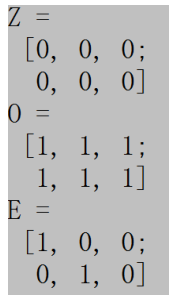## 实例

#include"opencv2/opencv.hpp"
#include<iostream>
using  namespace std;
using namespace cv;
int main() {
Mat src;
if (src.empty()) {
cerr << "open error" << endl;
return -1;
}

namedWindow("原图", WINDOW_AUTOSIZE);
imshow("原图", src);

//构造 Mat::Mat(Size size,int type)，没有数据
Mat dst;
dst = Mat(src.size(), src.type());
dst = Scalar(127, 0, 255);  //B=127 G=0 R=255
namedWindow("new1", WINDOW_AUTOSIZE);
imshow("new1", dst);

//和原图一模一样
//1. Mat.clone()
//和原图一模一样
Mat dst2;
dst2 = src.clone();
namedWindow("new2", WINDOW_AUTOSIZE);
imshow("new2", dst2);

//2. Mat.copyTo(output)
Mat dst3;
src.copyTo(dst3);
namedWindow("new3", WINDOW_AUTOSIZE);
imshow("new3", dst3);

//3. cvtColor构造图片
Mat dst4;
cvtColor(src,dst4, CV_BGR2GRAY);
cout << "dst4.cols=" << dst4.cols << endl ;
cout << "dst4.rows=" << dst4.rows << endl ;
cout << "src.channels=" << src.channels() << endl ;
cout << "dst4.channels=" << dst4.channels() << endl;
const uchar* p = dst4.ptr<uchar>(0);
cout << "dst4 first pixel value=" << &p << endl;
namedWindow("new4", WINDOW_AUTOSIZE);
imshow("new4", dst4);

//图片内容为空
//Mat::Mat(int rows, int cols, int type, void* data, size_t step=AUTO_STEP)
Mat dst5(3,3, CV_8UC3, Scalar(0, 0, 255));
cout << "dst5=" << endl << dst5 << endl;
namedWindow("new5", WINDOW_AUTOSIZE);
imshow("new5", dst5);

//Mat
Mat dst6;
dst6.create(src.size(), src.type());
dst6 = Scalar(0, 0, 255);
namedWindow("new6", WINDOW_AUTOSIZE);
imshow("new6", dst6);

//
Mat dst7;
dst7=Mat::zeros(src.size(), src.type());
namedWindow("new7", WINDOW_AUTOSIZE);
imshow("new7", dst7);

waitKey(0);
}


# 3、矩阵的基本元素表达

OpenCV 中有模板类 Vec，可以表示一个向量。OpenCV 中使用 Vec 类预定义了一些小向量，可以将之用于矩阵元素的表达。

typedef Vec<uchar, 3> Vec3b;
typedef Vec<uchar, 4> Vec4b;

typedef Vec<short, 2> Vec2s;
typedef Vec<short, 3> Vec3s;
typedef Vec<short, 4> Vec4s;

typedef Vec<int, 2> Vec2i;
typedef Vec<int, 3> Vec3i;
typedef Vec<int, 4> Vec4i;

typedef Vec<float, 2> Vec2f;
typedef Vec<float, 3> Vec3f;
typedef Vec<float, 4> Vec4f;
typedef Vec<float, 6> Vec6f;

typedef Vec<double, 2> Vec2d;
typedef Vec<double, 3> Vec3d;
typedef Vec<double, 4> Vec4d;
typedef Vec<double, 6> Vec6d;


• 8U 类型的 RGB 彩色图像可以使用 Vec3b，
• 3 通道 float 类型的矩阵可以使用 Vec3f。

Vec3b color; //用 color 变量描述一种 RGB 颜色
color=255; //B 分量
color=0; //G 分量
color=0; //R 分量


# 5、选取图像局部区域

Mat 类提供了多种方便的方法来选择图像的局部区域。使用这些方法时需要注意，这些方法并不进行内存的复制操作。如果将局部区域赋值给新的 Mat 对象，新对象与原始对象共用相同的数据区域，不新申请内存，因此这些方法的执行速度都比较快。

## 5.1、单行或单列选择

Mat  Mat::row(int i)  const
Mat  Mat::col  (int j)  const


Mat line=A.row(i);

A.row(j) = A.row(i)*2;

## 5.2、用Range选择多行或多列

Range 是 OpenCV 中新增的类，该类有两个关键变量 star 和 end。Range 对

class Range
{
public:
...
int start, end;
};


Range 类还提供了一个静态方法 all()，这个方法的作用如同 Matlab 中的“:”，表所有的行或者所有的列。

创建一个单位阵
Mat A=Mat::eye(10,10,CV_32S);

Mat B=A(Range::all(),Range(1,3));

Mat C = B(Range(5, 9), Range::all());
其实等价于 C = A(Range(5, 9), Range(1, 3))


## 5.3、 感兴趣区域

• 使用构造函数
//创建宽度为 320，高度为 240 的 3 通道图像
Mat img(Size(320,240),CV_8UC3);
//roi 是表示 img 中 Rect(10,10,100,100)区域的对象
Mat roi(img, Rect(10,10,100,100));
//使用构造函数
Mat roi4(img, Range(10,100),Range(10,100));

• 使用括号运算符
Mat roi2 = img(Rect(10,10,100,100));


 Mat roi3 = img(Range(10,100),Range(10,100));


## 5.4、取对角线元素

Mat Mat::diag(int d) const

1. 参数 d=0 时，表示取主对角线；
2. 当参数 d>0 是，表示取主对角线下方的次对角线，
如 d=1 时，表示取主对角线下方，且紧贴主多角线的元素；
3. 当参数 d<0 时，表示取主对角线上方的次对角线。
如同row()和col()函数，diag()函数也不进行内存复制操作，其复杂度也是O(1)。

# 6、Mat表达式

C = A + B + 1;

• 加法，减法，取负：A+B，A-B，A+s，A-s，s+A，s-A，-A
• 缩放取值范围：A*alpha
• 矩阵对应元素的乘法和除法： A.mul(B)，A/B，alpha/A
• 矩阵乘法：A*B （注意此处是矩阵乘法，而不是矩阵对应元素相乘）
• 矩阵转置：A.t()
• 矩阵求逆和求伪逆：A.inv()
• 矩阵比较运算：A cmpop B，A cmpop alpha，alpha cmpop A。
此处 cmpop可以是>，>=，==，!=，<=，<。如果条件成立，则结果矩阵（8U 类型矩阵）的对应元素被置为 255；否则置 0。
• 矩阵位逻辑运算：A logicop B，A logicop s，s logicop A，~A，
此处 logicop可以是&，|和^。
• 矩阵对应元素的最大值和最小值：min(A, B)，min(A, alpha)，max(A, B)，max(A, alpha)。
• 矩阵中元素的绝对值：abs(A)
• 叉积和点积：A.cross(B)，A.dot(B)
下面例程展示了 Mat 表达式的使用方法，例程的输出结果如图所示。
#include <iostream>
#include "opencv2/opencv.hpp"
using namespace std;
using namespace cv;
int main(int argc, char* argv[])
{
//创建了一个4*4的单位矩阵，单通道，32位
Mat A = Mat::eye(4, 4, CV_32SC1);
cout << "A=" << A << endl;
//单位矩阵的每个元素都是*3+1；
Mat B = A * 3 + 1;
//B矩阵的主对角线【4,4,4,4】与B矩阵的第二列元素【1,4,1,1】对应相加
Mat C = B.diag(0) + B.col(1);

//输出元素：
cout << "A = " << A << endl << endl;
cout << "B = " << B << endl << endl;
cout << "C = " << C << endl << endl;
cout << "A = " << A << endl << endl;
//C矩阵与B的主对角线的点积 5*4+8*4+5*4+5*4；
cout << "C .* diag(B) = " << C.dot(B.diag(0)) << endl;

return 0;
}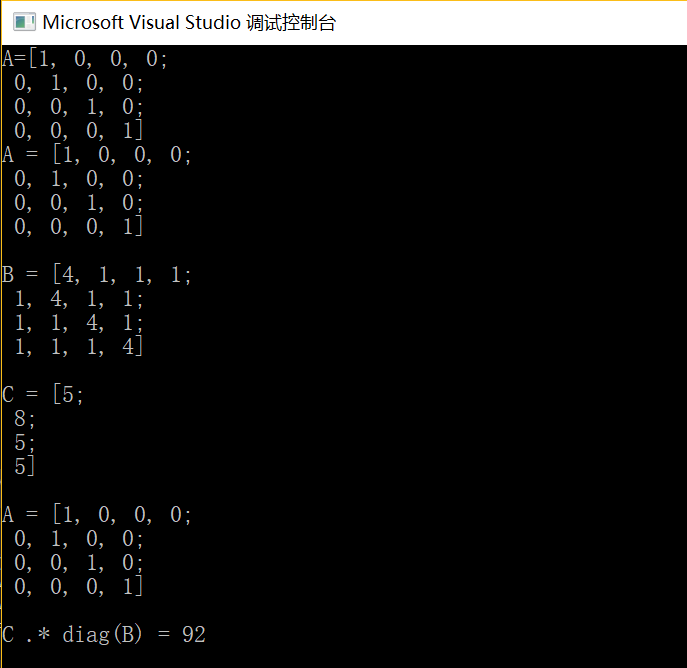# 7、Mat_类

Mat_类是对 Mat 类的一个包装，其定义如下：

template<typename _Tp> class Mat_ : public Mat
{
public:
//只定义了几个方法
//没有定义新的属性
};


Mat M(600, 800, CV_8UC1);
for( int i = 0; i < M.rows; ++i){
uchar * p = M.ptr<uchar>(i);
for( int j = 0; j < M.cols; ++j ){
double d1 = (double) ((i+j)%255);
M.at<uchar>(i,j) = d1;
double d2 = M.at<double>(i,j);//此行有错
}
}


#include <iostream>
#include "opencv2/opencv.hpp"
#include <stdio.h>
using namespace std;
using namespace cv;
int main(int argc, char* argv[])
{
Mat M(600, 800, CV_8UC1);
for (int i = 0; i < M.rows; ++i)
{
//获取指针时需要指定类型
uchar * p = M.ptr<uchar>(i);
for (int j = 0; j < M.cols; ++j)
{
double d1 = (double)((i + j) % 255);
//用 at()读写像素时，需要指定类型
M.at<uchar>(i, j) = d1;
//下面代码错误，应该使用 at<uchar>()
//但编译时不会提醒错误
//运行结果不正确，d2 不等于 d1
//double d2 = M.at<double>(i, j);
}
}

//在变量声明时指定矩阵元素类型
Mat_<uchar> M1 = (Mat_<uchar>&)M;
for (int i = 0; i < M1.rows; ++i)
{
//不需指定元素类型，语句简洁
uchar * p = M1.ptr(i);
for (int j = 0; j < M1.cols; ++j)
{

double d1 = (double)((i + j) % 255);
//直接使用 Matlab 风格的矩阵元素读写，简洁
M1(i, j) = d1;
double d2 = M1(i, j);
}
}
return 0;
}


# 8、Mat类的内存管理

Mat 是一个类，由两个数据部分组成：矩阵头（包含矩阵尺寸，存储方法，存储地址等信息）和一个指向存储所有像素值的矩阵的指针。矩阵头的尺寸是常数值，但矩阵本身的尺寸会依图像的不同而不同，通常比矩阵头的尺寸大数个数量级。复制矩阵数据往往花费较多时间，因此除非有必要，不要复制大的矩阵。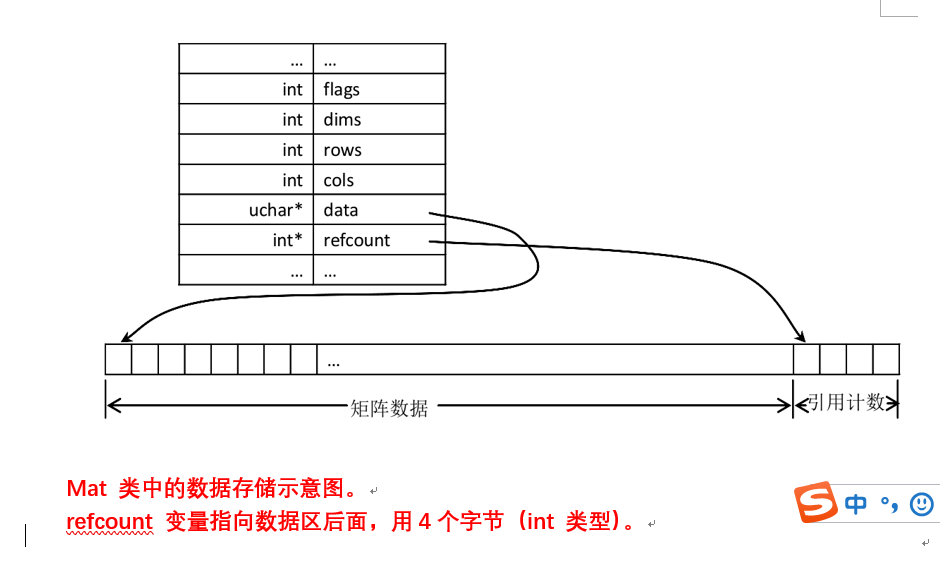Mat A(100,100, CV_8UC1);
Mat B = A;
Mat C = A(Rect(50,50,30,30));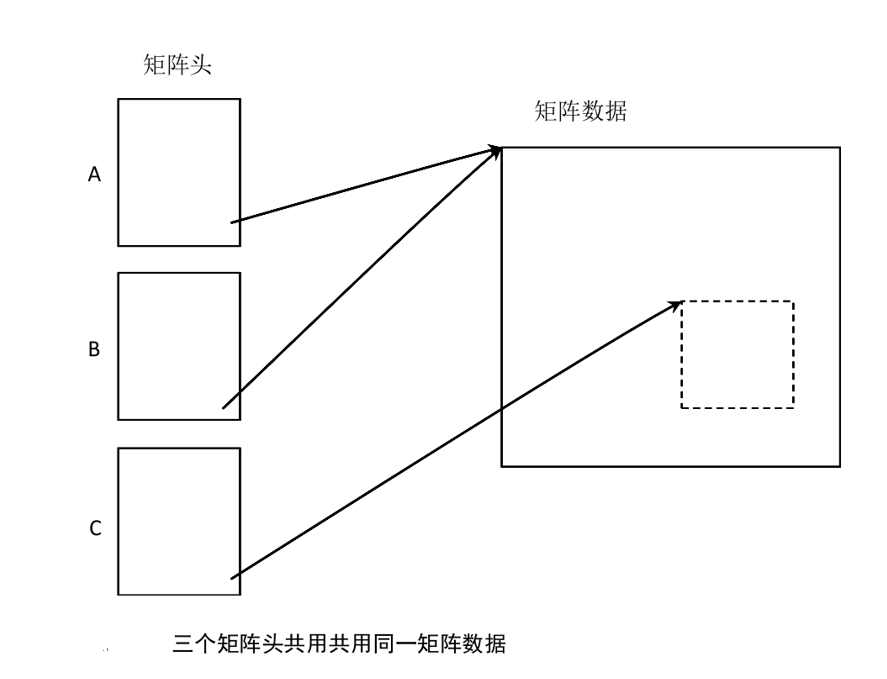# 9、输出

• 首先创建一个矩阵，并用随机数填充。填充的范围由 randu()函数的第二个参数和第三个参数确定，下面代码是介于 0 到 255 之间。

Mat R = Mat(3, 2, CV_8UC3);

randu(R, Scalar::all(0), Scalar::all(255));

#### 默认格式输出的代码如下：

cout << "R (default) = " << endl << R << endl << endl;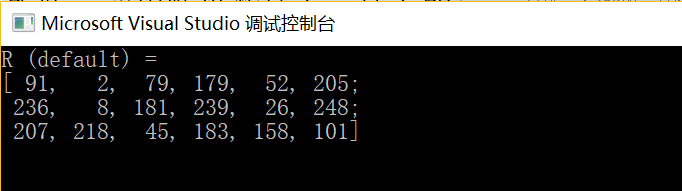#### python 格式输出的代码如下：

//cout << "r(Python风格_openCV2) = " << format(r, “python”) << “;” << endl << endl;
cout << "r(Python风格_openCV3) = " << endl << format(r, Formatter::FMT_PYTHON) << endl << endl;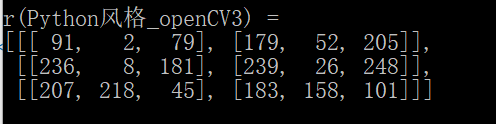#### 以逗号分割的输出的代码如下：

//cout << "r(,分隔风格_openCV2) = " << format(r, “csv”) << “;” << endl << endl;
cout << "r(,分隔风格_openCV3) = " << endl << format(r, Formatter::FMT_CSV) << endl << endl;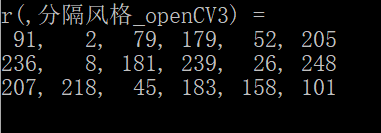#### numpy 格式输出的代码如下：

//cout << "r(numpy风格_openCV2) = " << format(r, “numpy”) << “;” << endl << endl;
cout << "r(numpy风格_openCV3) = " << endl << format(r, Formatter::FMT_NUMPY)<< endl << endl;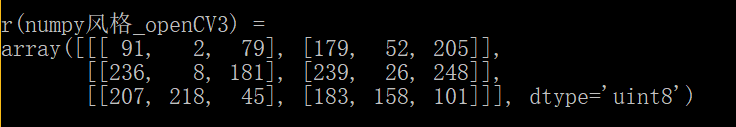#### C 语言格式输出的代码如下：

// cout << "r(C语言风格_openCV2) = " << format(r, “c”) << “;” << endl << endl;
cout << "r(C语言风格_openCV3) = " << endl << format(r, Formatter::FMT_C) << endl << endl;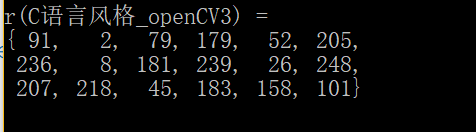• 除了 Mat 对象可以使用<<符号输出，其他的很多类型也支持<<输出。

Point2f P(5, 1);
cout << "Point (2D) = " << P << endl << endl;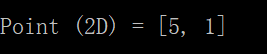Point3f P3f(2, 6, 7);
cout << "Point (3D) = " << P3f << endl << endl;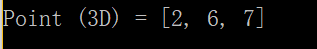# 10、Mat与IpIImage和CvMat的转换

## 10.1、Mat转为IpIImage和CvMat格式

void mycvOldFunc(IplImage * p, …);

Mat img(Size(320, 240), CV_8UC3);
···
IplImage iplimg = img; //转为 IplImage 结构
mycvOldFunc( & iplimg, ...);//对 iplimg 取地址


CvMat cvimg = img; //转为 CvMat 结构

## 10.2、IpIImage和CvMat格式转化为Mat

Mat 类有两个构造函数，可以实现 IplImage 和 CvMat 到 Mat 的转换。这两个函数都有一个参数copyData。如果copyData的值是false，那么Mat将与IplImage或 CvMat 共用同一矩阵数据；如果值是 true，Mat 会新申请内存，然后将 IplImage或 CvMat 的数据复制到 Mat 的数据区。

Mat::Mat(const CvMat* m, bool copyData=false)
Mat::Mat(const IplImage* img, bool copyData=false)


IplImage * iplimg = cvLoadImage("lena.jpg");
Mat im(iplimg, true);


©️2019 CSDN 皮肤主题: 终极编程指南 设计师: CSDN官方博客